# trigonometry

(redirected from Trignometry)
Also found in: Dictionary, Thesaurus.

## trigonometry

[Gr.,=measurement of triangles], a specialized area of geometry concerned with the properties of and relations among the parts of a triangle. Spherical trigonometry is concerned with the study of triangles on the surface of a sphere rather than in the plane; it is of considerable importance in surveying, navigation, and astronomy.

### The Basic Trigonometric Functions

Trigomometry originated as the study of certain mathematical relations originally defined in terms of the angles and sides of a right triangle, i.e., one containing a right angle (90°). Six basic relations, or trigonometric functions, are defined.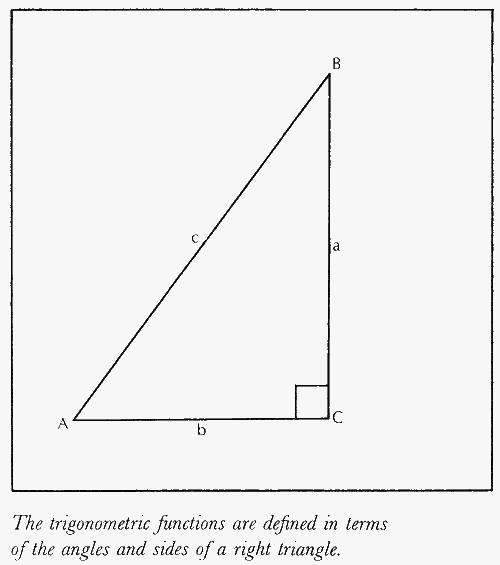If A, B, and C are the measures of the angles of a right triangle (C=90°) and a, b, and c are the lengths of the respective sides opposite these angles, then the six functions are expressed for one of the acute angles, say A, as various ratios of the opposite side (a), the adjacent side (b), and the hypotenuse (c), as set out in the table.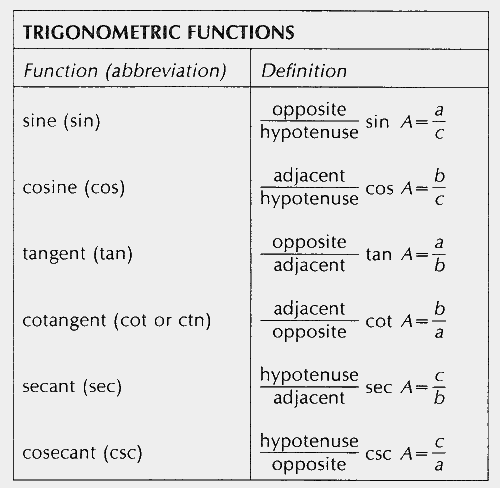Although the actual lengths of the sides of a right triangle may have any values, the ratios of the lengths will be the same for all similar right triangles, large or small; these ratios depend only on the angles and not on the actual lengths. The functions occur in pairs—sine and cosine, tangent and cotangent, secant and cosecant—called cofunctions. In equations they are usually represented as sin, cos, tan, cot, sec, and csc. Since in ordinary (Euclidean) plane geometry the sum of the angles of a triangle is 180°, angles A and B must add up to 90° and therefore are complementary angles. From the definitions of the functions, it may be seen that sin B=cos A, cos B=sin A, tan B=cot A, and sec B=csc A; in general, the function of an angle is equal to the cofunction of its complement. Since the hypotenuse (c), is always the longest side of a right triangle, the values of the sine and cosine are always between zero and one, the values of the secant and cosecant are always equal to or greater than one, and the values of the tangent and cotangent are unbounded, increasing from zero without limit.

For certain special right triangles the values of the functions may be calculated easily; e.g., in a right triangle whose acute angles are 30° and 60° the sides are in the ratio 1 :√3 : 2, so that sin 30°=cos 60°=1/2, cos 30°=sin 60°=√3/2, tan 30°=cot 60°=1/√3, cot 30°=tan 60°=√3, sec 30°=csc 60°=2/√3, and csc 30°=sec 60°=2. For other angles, the values of the trigonometric functions are usually found from a set of tables or a scientific calculator. For the limiting values of 0° and 90°, the length of one side of the triangle approaches zero while the other approaches that of the hypotenuse, resulting in the values sin 0°=cos 90°=0, cos 0°=sin 90°=1, tan 0°=cot 90°=0, and sec 0°=csc 90°=1; since division by zero is undefined, cot 0°, tan 90°, csc 0°, and sec 90° are all undefined, having infinitely large values.

A general triangle, not necessarily containing a right angle, can also be analyzed by means of trigonometry, and various relationships are found to exist between the sides and angles of the general triangle. For example, in any plane triangle a/sin A=b/sin B=c/sin C. This relationship is known as the Law of Sines. The related Law of Cosines holds that a2=b2+c2−2bc cosA and the Law of Tangents holds that (ab)/(a+b)=[tan 1-2(AB)]/[tan 1-2(A+B)]. Each of the trigonometric functions can be represented by an infinite seriesseries,
in mathematics, indicated sum of a sequence of terms. A series may be finite or infinite. A finite series contains a definite number of terms whose sum can be found by various methods. An infinite series is a sum of infinitely many terms, e.g.
.

### Extension of the Trigonometric Functions

The notion of the trigonometric functions can be extended beyond 90° by defining the functions with respect to Cartesian coordinatesCartesian coordinates
[for René Descartes], system for representing the relative positions of points in a plane or in space. In a plane, the point P is specified by the pair of numbers (x,y
. Let r be a line of unit length from the origin to the point P (x,y), and let θ be the angle r makes with the positive x-axis. The six functions become sin θ =y/r=y, cos θ=x/r=x, tan θ=y/x, cot θ=x/y, sec θ=r/x=1/x, and csc θ=r/y=1/y. As θ increases beyond 90°, the point P crosses the y-axis and x becomes negative; in quadrant II the functions are negative except for sin θ and csc θ. Beyond θ=180°, P is in quadrant III, y is also negative, and only tan θ and cot θ are positive, while beyond θ=270° P moves into quadrant IV, x becomes positive again, and cos θ and sec θ are positive.Since the positions of r for angles of 360° or more coincide with those already taken by r as θ increased from 0°, the values of the functions repeat those taken between 0° and 360° for angles greater than 360°, repeating again after 720°, and so on.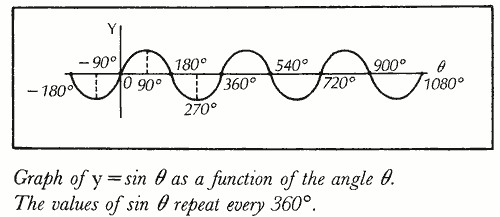This repeating, or periodic, nature of the trigonometric functions leads to important applications in the study of such periodic phenomena as light and electricity.

The following article is from The Great Soviet Encyclopedia (1979). It might be outdated or ideologically biased.

## Trigonometry

the branch of mathematics in which trigonometric functions and their applications to geometry are studied. Trigonometry is divided into plane trigonometry and spherical trigonometry. The theory of trigonometric functions and its applications to the solution of plane right and oblique triangles are studied in secondary school.

Consider a plane triangle with sides a, b, and c and opposite angles A, B, and C. It can be shown that A + B + C = π. Suppose the altitudes of the triangle are ha, hb, and hc; the perimeter is 2p; the area is S; and the diameter of a circle circumscribed about the triangle is 2R. The following are fundamental relations of plane trigonometry:

(1) Law of sines: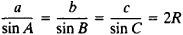(2) Law of cosines:

a2 = b2 + c2 –2bc cos A

(3) Law of tangents: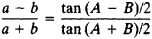(4) Formula for the area of a triangle:If the sides of a triangle are known, the angles can be found from the law of cosines or from equations of the form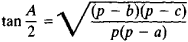Although the development of plane trigonometry began later than that of spherical trigonometry, some of the theorems of plane trigonometry were known in earlier times. For example, the 12th and 13th theorems of the second book of Euclid’s Elements (third century B.C.) essentially express the law of cosines. Important contributions to the development of plane trigonometry were made by al-Battani (second half of the ninth and early tenth centuries), Abu al-Wafa (tenth century), Bhaskara (12th century), and Nasir al-Din al-Tusi (13th century), who were aware of the law of sines. The law of tangents was derived by Re-giomontanus (15th century). Further contributions were made by Copernicus (first half of the 16th century), T. Brahe (second half of the 16th century), F. Vieta (16th century), and J. Kepler (late 16th and first half of the 17th centuries). Trigonometry assumed its modern form in the work of L. Euler (18th century).

### REFERENCE

Kochetkov, E. S., and E. S. Kochetkova. Algebra i elementarnye funktsii, parts 1–2. Moscow, 1966.

## trigonometry

[‚trig·ə′näm·ə·trē]
(mathematics)
The study of triangles and the trigonometric functions.
McGraw-Hill Dictionary of Scientific & Technical Terms, 6E, Copyright © 2003 by The McGraw-Hill Companies, Inc.

## trigonometry

the branch of mathematics concerned with the properties of trigonometric functions and their application to the determination of the angles and sides of triangles. Used in surveying, navigation, etc.
Collins Discovery Encyclopedia, 1st edition © HarperCollins Publishers 2005
Site: Follow: Share:
Open / Close